# Direct Current ( DC )

### What is a direct current (DC) ?

Direct current (DC) is an electric current that flows continuously in one direction only. This is the type of electrical current is supplied if the voltaic cell. As long as the polarity of the voltage at the bases voltaic cell is fixed and does not reverse, the voltaic cell can be referred to as a source of direct voltage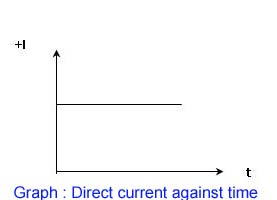Sources of Direct Current

1) Battery

a source of direct current which is designed to produce output voltage and maximum output current of a certain.

2) Solar

is a source of direct current in which the rays of the sun can produce electricity

3) DC rectifier

is a device to convert from alternating current to direct current consistently

Circuit Connection

1) Series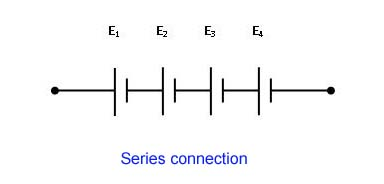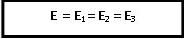In order of series,
The total sum of the output voltage = voltage of the cell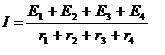Current Output

In the current (I) the product flow in each cell are the same.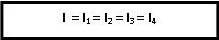Total resistance = sum of the individual resistance of the cell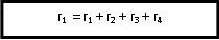2) Parallel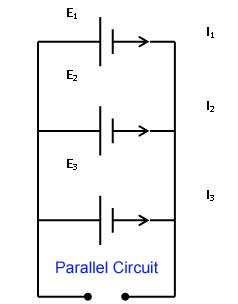In the parallel arrangement, Output voltage is the same.Current output = sum of currents of each cell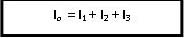Total resistance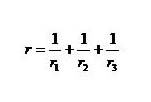3) Series-parallel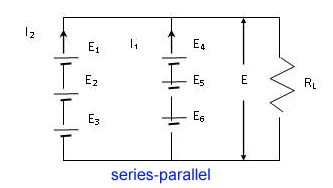Base Voltage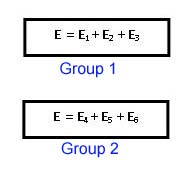Current OutputThis site uses Akismet to reduce spam. Learn how your comment data is processed.